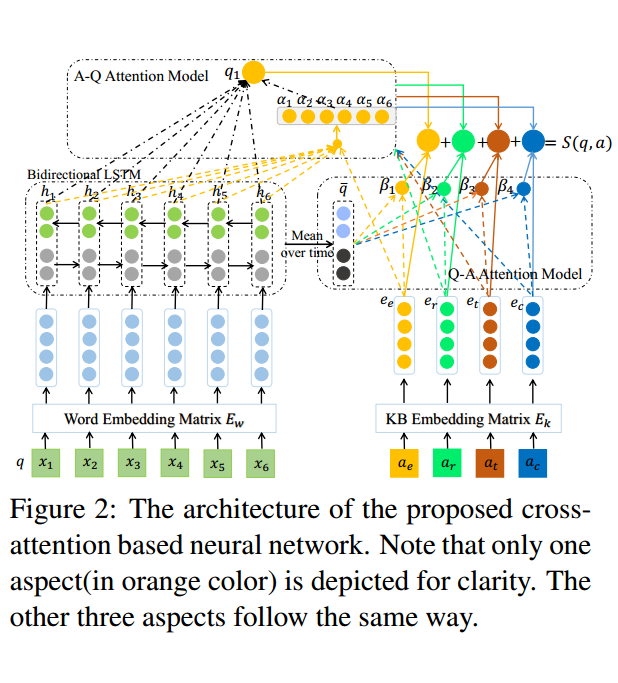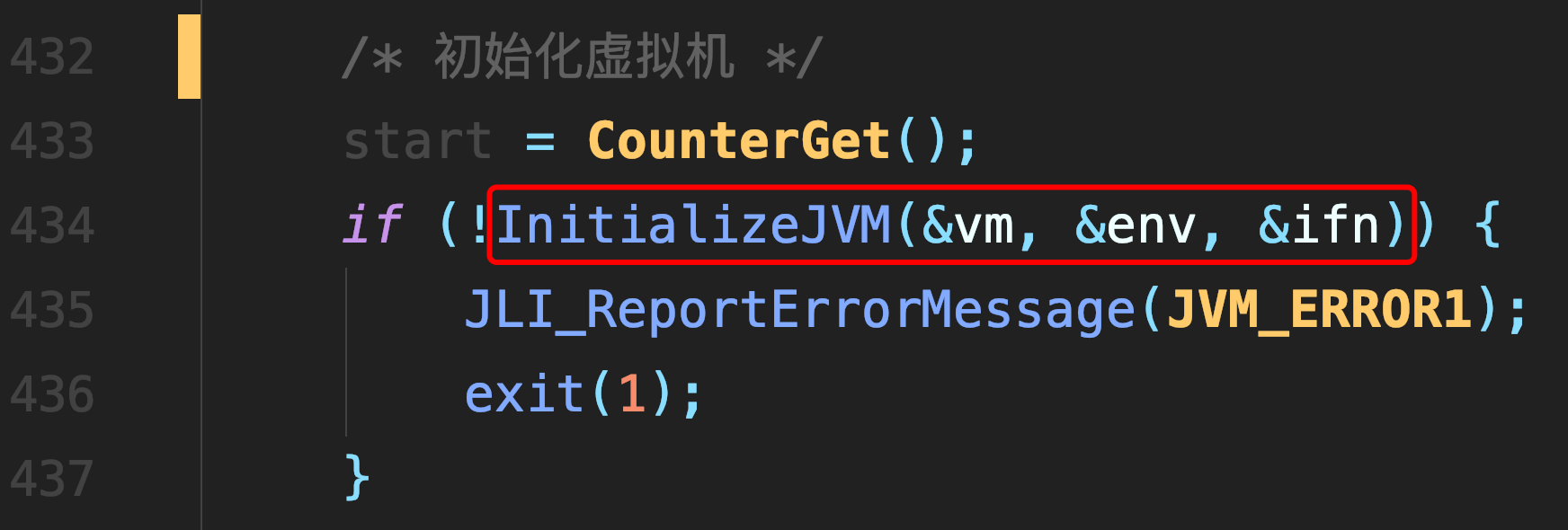# prim算法

### prim算法

• 输入：一个加权连通图，其中顶点集合为V，边集合为E；
• 初始化：Vnew = {x}，其中x为集合V中的任一节点（起始点），Enew = {},为空；
• 重复下列操作，直到Vnew = V：
• 在集合E中选取权值最小的边(u,v)其中u为集合Vnew中的元素，而v不在Vnew集合当中，并且v∈V（如果存在有多条满足前述条件即具有相同权值的边，则可任意选取其中之一）；
• 将v加入集合Vnew中，将(u, v)边加入集合Enew中；
• 输出：使用集合Vnew和Enew来描述所得到的最小生成树此为原始的加权连通图。每条边一侧的数字代表其权值。 - - -顶点D被任意选为起始点。顶点ABEF通过单条边与D相连。A是距离D最近的顶点，因此将A及对应边AD以高亮表示。 C,G A,B,E,F D下一个顶点为距离DA最近的顶点。BD为9，距A为7，E为15，F为6。因此，FDA最近，因此将顶点F与相应边DF以高亮表示。 C,G B,E,F D,A算法继续重复上面的步骤。距离A为7的顶点B被高亮表示 C B,E,G D,A,F在当前情况下，可以在CEG间进行选择。CB为8，EB为7，GF为11。点E最近，因此将顶点E与相应边BE高亮表示。 - C,E,G D,A,F,B这里，可供选择的顶点只有CGCE为5，GE为9，故选取C，并与边EC一同高亮表示。 - C,G D,A,F,B,E顶点G是唯一剩下的顶点，它距F为11，距E为9，E最近，故高亮表示G及相应边EG - G D,A,F,B,E,C现在，所有顶点均已被选取，图中绿色部分即为连通图的最小生成树。在此例中，最小生成树的权值之和为39。 - - D,A,F,B,E,C,G

### C++代码

``````#include<iostream>
using namespace std;
#include<limits>
#include<map>
#define size 7

int a[size][size] = {
{0, 7, INT_MAX, 5, INT_MAX, INT_MAX, INT_MAX},
{7, 0, 8, 9, 7, INT_MAX, INT_MAX},
{INT_MAX, 8, 0, INT_MAX, 5, INT_MAX, INT_MAX},
{5, 9, INT_MAX, 0, 15, 6, INT_MAX},
{INT_MAX, 7, 5, 15, 0, 8, 9},
{INT_MAX, INT_MAX, INT_MAX, 6, 8, 0, 11},
{INT_MAX, INT_MAX, INT_MAX, INT_MAX, 9, 11, INT_MAX}
};

char change(int i) {
switch(i){
case 0: return 'A';
case 1: return 'B';
case 2: return 'C';
case 3: return 'D';
case 4: return 'E';
case 5: return 'F';
case 6: return 'G';

}
return 'O';
}
int main() {
bool visited[size];
for(int i = 0; i < size; i++) visited[i] = false;

visited = true; /*A为起始点*/

int distance[size];
map<int, int> M; /*记录加入边记录*/
int count = 0; /*最小生成树大小*/

/*初始化距离*/
for(int i = 1; i < size; i++)  {
distance[i] = a[i];
M[i] = 0;
}

/*找到n-1个点*/
for(int i = 1; i < size; i++) {

int min = INT_MAX;
int w = 0;
for(int j = 0; j < size; j++) {
if(visited[j]) continue;
if(distance[j] < min) {
min = distance[j];
w = j;
}
}
count += min;
visited[w] = true;
cout << "第" << i << "条边: " << change(M[w])<< "->" << change(w)<< endl;

/*更新距离*/
for(int j = 1; j < size; j++){
if(visited[j]) continue;
if(a[w][j] < distance[j]) {
distance[j] = a[w][j];
M[j] = w;
}
}
}
cout << "最小生成树大小: " << count << endl;
}
/* 第1条边: A->D 第2条边: D->F 第3条边: A->B 第4条边: B->E 第5条边: E->C 第6条边: E->G 最小生成树大小: 39 */``````

##### 热门推荐
•浏览(655)
•浏览(565)
•浏览(548)
•浏览(488)
•浏览(434)
•浏览(424)
•浏览(381)
•浏览(367)
•浏览(362)
•浏览(357)